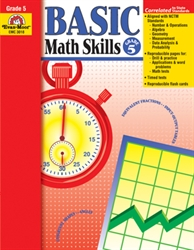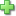# Basic Math Skills 5

Current Retail Price: \$29.99
Not in stock

See series description for full review.

In Basic Math Skills, Grade 5, you’ll find just what you need to provide extra skill practice for individual students, small groups, or the whole class. These reproducible pages are perfect for homework too.

Skills practiced include:

Number and Operations

• Compare and order positive rational numbers; use place value and rounding
• Compute with whole
• Identify odd, even, prime and composite numbers; identify factors and multiples
• Compute with fractions
• Compute with decimals
• Identify equivalent values and compare sets and values

Algebra

• Describe and extend numerical patterns
• Complete a function table (input/output table) with two operations
• Write rules for a function table in the form of an expression
• Locate points (including fractions and decimals) on a number line
• Locate and plot ordered pairs on a coordinate graph (first quadrant only)

Geometry

• Identify and construct two-dimensional blueprints (nets) of three-dimensional figures
• Identify congruent shapes using transformational geometry (rotations, translations, reflections)
• Identify lines of symmetry in two-dimensional figures

Measurement

• Identify and order metric measurements
• Identify, compare, and use customary and metric units of linear measurement
• Find conversions between units within a system of linear measurement
• Solve problems related to the calendar
• Read a thermometer and solve problems related to temperature
• Measure angles using a protractor, and classify angles as acute, obtuse, or right
• Find perimeter of polygons
• Find area of rectangles and squares
• Estimate and calculate volumes of rectangular prisms

Data Analysis and Probability

• Construct bar graphs, double bar graphs, line graphs, circle graphs, and stem and leaf plots
• Interpret bar graphs, double bar graphs, line graphs, circle graphs, and stem and leaf
• Analyze data utilizing a range, mean, median, and mode
• Compute theoretical probabilities for simple chance events
• Utilize counting techniques, tree charts, and organized lists to determine all possible combinations
Did you find this review helpful?
Series Description
Related Categories
Recommended for...
Customer ReviewsClick here to write a review